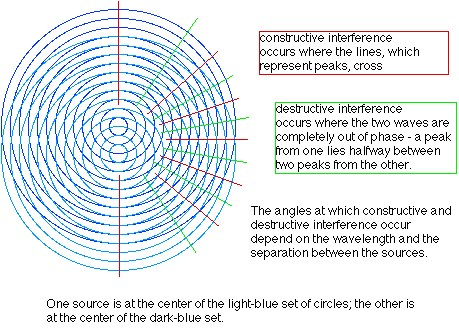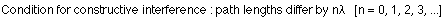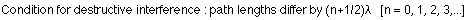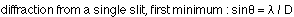## Diffraction

3-5-99

Section 11.13

### The interference of two waves of the same frequency

When two (or more) waves of the same frequency interfere, a variety of different results can be obtained. Consider first the special case of two sources separated by a small distance d, sending out waves of the same frequency. The sources are in phase with each other. For a particular separation and wavelength, the pattern is as shown in the diagram, with constructive interference taking place at certain angles and destructive interference taking place at other angles.When the sources send out waves in phase, constructive interference will occur at a particular point if the path lengths from the two sources to that point differ by an integral number of wavelengths.Destructive interference occurs at a particular point if the path lengths from the two sources to that point differ by an integral number of wavelengths + 1/2 a wavelength.### Diffraction

Diffraction is the bending of waves that takes place when the wave encounters openings or obstacles. The most interesting cases (i.e., the ones with interesting patterns of maxima and minima) are those in which the size of the openings or obstacles is about the same as the wavelength of the wave.

Interference, both constructive and destructive, is important to understanding why diffraction occurs. If the opening is divided into many small pieces, each piece can be thought of as an emitter of the wave. The waves from each piece of the opening are sent out in phase with each other; at some places they will interfere constructively, and at others they will interfere destructively.

Consider a point that is half a wavelength further from the center of the opening than from one side of the opening. This is the condition for destructive interference: the wave from the side of the opening will interfere destructively with the wave from the center of the opening. Similarly, the wave from the part of the opening next to the side will interfer destructively with the part of the opening next to the center, and so on - the waves from one half of the opening completely cancel the waves from the other half. It turns out that the points where this destructive interference occurs are all along one line, at an angle (measured from a line perpendicular to the opening) given by:If D, the width of the opening, is less than the wavelength than there is no place where the interference is completely destructive. If D is greater than the wavelength there is at least one angle where destructive interference occurs; the diffraction patterns in such cases are similar to the interference patterns produces by two sources close together.